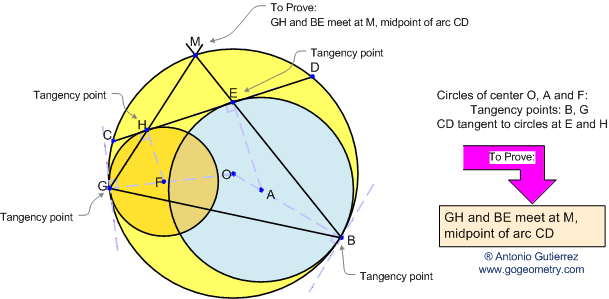< PREVIOUS PROBLEM  |  NEXT PROBLEM > Problem 54. Tangent Circles, angle bisector, midpoint. Level: High School, SAT Prep, College In the figure below, the circles of centers O and A are tangent at B, the circles O and F are tangent at G. A chord CD of the circle O is tangent to the circle A at E and tangent to the circle F at H. GH and BE meet at a point M. Prove that M is the midpoint of arc CD.Hints: See Problem 53   I have used Geometry Expressions to visualize these geometric forms and check out a variety of conjectures. Geometry Expressions is the world's first Interactive Symbolic Geometry System. This means: Geometric figures can be defined by either Symbolic Constraints or numeric locations.
 Home | Geometry | Problems | Open Problems | 10 Problems | Spanish Problem 54 | 51-60 | Circle Tangent Line | Email | By Antonio Gutierrez